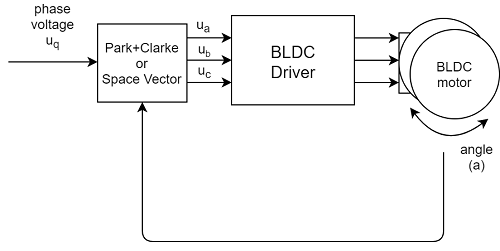# Torque control using voltage v2.0.2

In this library we are using the voltage as a substitute for the torque control, why is that and how does it work exactly?

## How does this work exactlyThe voltage control algorithm reads the angle a from the position sensor and the gets target Uq voltage value from the user and using the FOC algorithm sets the appropriate ua, ub and uc voltages to the motor. FOC algorithm ensures that these voltages generate the magnetic force in the motor rotor exactly with 90 degree offset from its permanent magnetic field, which guarantees maximal torque, this is called commutation.

This is a hard way of achieving exactly the principle of the DC motor. Because, for DC motors, the 90 degree angle in between generated magnetic field in rotor and the permanent magnetic field of the stator is performed in hardware, making it trivial for end-user. Now when you have the 90 degree constraint ensured by software (FOC algorithm) you can use this motor as any other voltage controlled DC motor.

Therefore as for the DC motor we know that the motor torque T is directly proportional to the current I:

T = I*K


Where K is the motor constant defined by its hardware. And we also know that the current is proportional to the set voltage U:

I  = (U - EMF)/R


Where R is the motor resistance and EMF is the generated back EMF voltage. This equation doesn’t take in consideration any dynamics but in general it works well enough.

So what we can conclude from all this is that (if we neglect the EMF):

T ~ I ~ U


This means that the torque is proportional to the current and since the current is proportional to the voltage then torque is proportional to the voltage as well.

Constrains of this approach: Beware!

This assumption of proportionality only works for statics not for dynamics, which means that we will have some current peaks due to different dynamical effects. But if the currents are not too large these effects can be neglected. Currents < 5A
For real torque control loop we will need to measure the current, but the hardware for current measurement is not really common for low-power applications making this kind of torque control implementation the only way to get around it.